# 基于光纤网的光缆线路故障告警技术研究The Study of Optical Line Fault Alarm Technology Based on Fiber Optic Network

DOI: 10.12677/SG.2018.81010, PDF, HTML, XML, 下载: 801  浏览: 2,847

Abstract: Yunnan terrain climate is complicated; cable lines often exist security risks. The normal operation of optical fiber cable is an important condition to ensure the safe and stable operation of power system. To grasp the real-time operating status of optical fiber lines in time is the basic prerequisite for ensuring the smooth flow of power system information. At present, the existing types of optical cable lines need to be managed and maintained, the topology is complicated, and the operation and maintenance tasks are difficult and heavy. This paper first builds an optical fiber network intelligent monitoring system architecture, which consists of management layer, monitoring layer and testing layer, which can realize the real-time monitoring of the operating status of the backbone optical cable network. Then, the optical fiber network OTDR detection technology is researched and a fiber optic cable fault alarm monitoring system including sensing subsystem, data acquisition and processing subsystem, expert and distance measuring subsystem is established. With the combination of positioning technology, the accurate positioning of optical fiber cable fault can be realized. And the fault point positioning segmentation function algorithm is used to analysis fiber optic cable fault and abnormal information, which improves operational efficiency and makes a contribution for building a modern, informative and intelligent power grid.

1. 引言

2. 光纤网光缆线路监测系统架构

3. 光纤网OTDR检测技术研究

${C}_{G}\left(z\right)=\underset{n=1}{\overset{N}{\sum }}{C}_{n}\left(z\right)=\underset{n=1}{\overset{N}{\sum }}{A}_{n}\mathrm{exp}\left[-\frac{4{\left(z-{u}_{n}\right)}^{2}}{{W}_{n}^{2}}\right]$ (1)

${\zeta }_{G}\left(\Delta \beta \right)={|{\int }_{-\infty }^{+\infty }{C}_{G}\left(z\right){\text{e}}^{-j\Delta \beta z}\text{d}z|}^{2}\approx \frac{\text{π}}{4}N{A}_{0}\mathrm{exp}\left[-{\left(\Delta \beta \right)}^{2}{W}_{0}^{2}/8\right]$ (2)

${L}_{G}=3.06\frac{1}{\Delta n}N{A}_{0}^{2}{W}_{0}\mathrm{exp}\left[-\Delta n\frac{{W}_{0}^{2}}{{d}^{2}}\right]$ (3)

OTDR是基于测量后向瑞利散射光信号的实用化测量仪器。利用OTDR可以方便地从单端对光纤进行非破坏性的测量，它能连续显示整个光纤线路的损耗相对于距离的变化。OTDR测试是通过将光脉冲注入到光纤中，当光脉冲在光纤内传输时，会由于光纤本身的性质、连接器、接头、弯曲或其它类似的事件而产生散射、反射，其中一部分的散射光和反射光经过同样的路径延时返回到OTDR中。OTDR根据入射信号与其返回信号的时间差τ，利用式1就可计算出上述事件点与OTDR的距离  。

$d=\frac{c\tau }{2n}$ (4)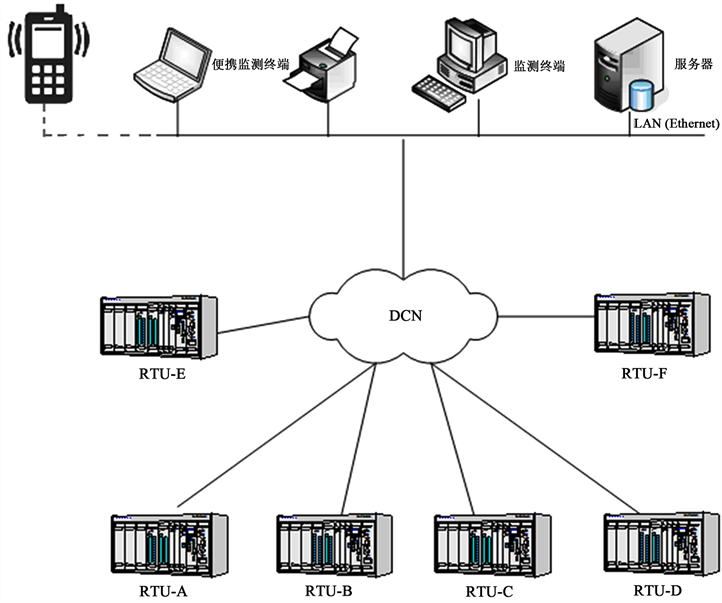Figure 1. The system architecture of optical fiber network monitoring

4. 光缆故障告警监测系统

4.1. 传感子系统

4.2. 专家及测距子系统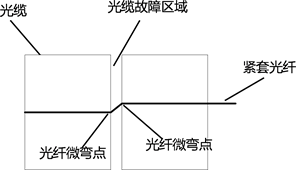Figure 2. The mechanism diagram of cable fault monitoring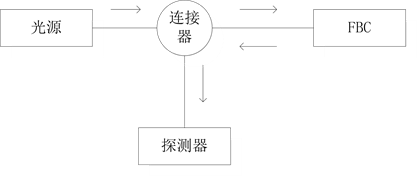Figure 3. FBG sensor subsystem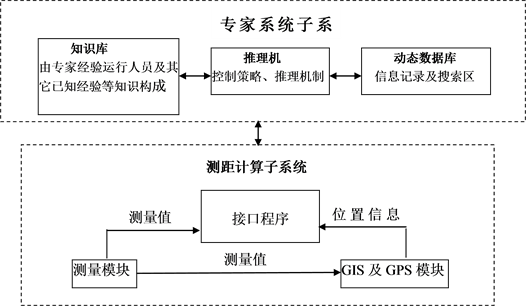Figure 4. The subsystem of expert and ranging

4.3. 光缆故障告警监测系统Figure 5. The monitoring system diagram of cable fault alarm

1) 光缆自动测试功能：自动实现光缆点名测试、周期测试，将结果与光缆特性库中的标准数据进行对比分析处理。按缆、段、站、纤、波长、方向、时间分析数据自动入库，自动分析全程衰耗、分段衰耗、接头衰耗和长度衰耗；自动判决当超过设置门限值时，按缆、段、纤分级告警，逐级自动备份所有监控参数数据库和告警记录数据。

2) 光功率监测系统功能：通过实时在线监测光纤收光功率，根据可修改的报警门限值为依据，进行判断，当收光功率变化超常时，将以不同级别输出告警信息。并将触发光缆自动测试子系统工作，启动 OTDR进行故障点位置判断。

3) 光缆运行维护管理功能：光缆运行统计分析管理功能生成光缆故障、光纤故障、光纤资源管理、监测系统设备等报表；提供可闻、可视(图形和文本)告警指示，显示当前告警事件和历史告警事件，并可对告警、事件进行分类保存和管理，灵活查询和统计、分析，形成统计报表；能够对故障按线路、光缆、路由、电路等统计月报表；当受理障碍信息时，能发出障碍通知单，启动光缆检修流程。

4) 光缆资源管理功能：按照国网公司标准命名规范和通信系统管理系统统一格式建立各种统计报表模型，建立光缆各个光纤配线节点、光缆接头盒位置、光传输设备和业务相关通信方式记录，并进行编辑、创建、查询、显示、打印等。

5. 故障测距算法及测试技术研究

5.1. 故障点定位分段函数算法

$L=\underset{m}{\sum }{L}_{m}\left(1+k\right)+a\left(m-1\right)$ (5)Table 1. The data table of fiber optic cable reference point

$y=\left\{\begin{array}{l}{y}_{0}\text{\hspace{0.17em}}\text{\hspace{0.17em}}\text{\hspace{0.17em}}\text{\hspace{0.17em}}\text{\hspace{0.17em}}\text{\hspace{0.17em}}\text{\hspace{0.17em}}\text{\hspace{0.17em}}\text{\hspace{0.17em}}\text{\hspace{0.17em}}\text{\hspace{0.17em}}\text{\hspace{0.17em}}\text{\hspace{0.17em}}\text{\hspace{0.17em}}\text{\hspace{0.17em}}\text{\hspace{0.17em}}\text{\hspace{0.17em}}\text{\hspace{0.17em}}\text{\hspace{0.17em}}\text{\hspace{0.17em}}\text{\hspace{0.17em}}\left(0\le x<{x}_{0}\right)\\ {y}_{0}+{f}_{1}\left(x\right)\text{\hspace{0.17em}}\text{\hspace{0.17em}}\text{\hspace{0.17em}}\text{\hspace{0.17em}}\text{\hspace{0.17em}}\text{\hspace{0.17em}}\text{\hspace{0.17em}}\text{\hspace{0.17em}}\text{\hspace{0.17em}}\text{ }\text{ }\left({x}_{0}\le x<{x}_{1}\right)\\ {y}_{1}\text{\hspace{0.17em}}\text{\hspace{0.17em}}\text{\hspace{0.17em}}\text{\hspace{0.17em}}\text{\hspace{0.17em}}\text{\hspace{0.17em}}\text{\hspace{0.17em}}\text{\hspace{0.17em}}\text{\hspace{0.17em}}\text{\hspace{0.17em}}\text{\hspace{0.17em}}\text{\hspace{0.17em}}\text{\hspace{0.17em}}\text{\hspace{0.17em}}\text{\hspace{0.17em}}\text{\hspace{0.17em}}\text{\hspace{0.17em}}\text{\hspace{0.17em}}\text{\hspace{0.17em}}\text{\hspace{0.17em}}\text{\hspace{0.17em}}\left({x}_{1}\le x<{x}_{2}\right)\\ {y}_{1}+{f}_{3}\left(x\right)\text{\hspace{0.17em}}\text{\hspace{0.17em}}\text{\hspace{0.17em}}\text{\hspace{0.17em}}\text{\hspace{0.17em}}\text{\hspace{0.17em}}\text{\hspace{0.17em}}\text{\hspace{0.17em}}\text{\hspace{0.17em}}\text{\hspace{0.17em}}\left({x}_{2}\le x<{x}_{3}\right)\\ \text{\hspace{0.17em}}\text{\hspace{0.17em}}\text{\hspace{0.17em}}\text{\hspace{0.17em}}\text{\hspace{0.17em}}\text{\hspace{0.17em}}\text{\hspace{0.17em}}\text{\hspace{0.17em}}\text{\hspace{0.17em}}\text{\hspace{0.17em}}\text{\hspace{0.17em}}\text{\hspace{0.17em}}\text{\hspace{0.17em}}\text{\hspace{0.17em}}⋮\\ {y}_{m-2}+{f}_{m}\left(x\right)\text{\hspace{0.17em}}\text{\hspace{0.17em}}\text{\hspace{0.17em}}\text{\hspace{0.17em}}\text{\hspace{0.17em}}\left({x}_{m-2}\le x<{x}_{m-1}\right)\\ {y}_{m-1}\left(x\right)\text{\hspace{0.17em}}\text{\hspace{0.17em}}\text{\hspace{0.17em}}\text{\hspace{0.17em}}\text{\hspace{0.17em}}\text{\hspace{0.17em}}\text{\hspace{0.17em}}\text{\hspace{0.17em}}\text{\hspace{0.17em}}\text{\hspace{0.17em}}\text{\hspace{0.17em}}\text{\hspace{0.17em}}\text{\hspace{0.17em}}\left({x}_{m-1}\le x<{x}_{m}\right)\end{array}$

5.2. 测试技术研究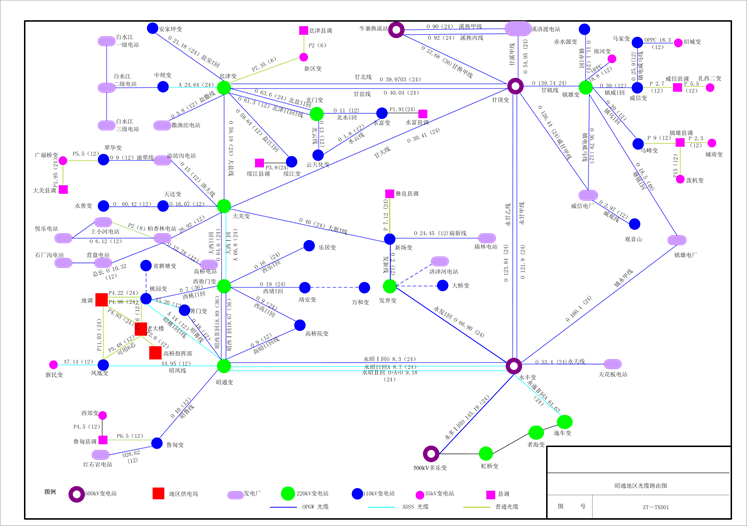Figure 6. The layout of test point optimization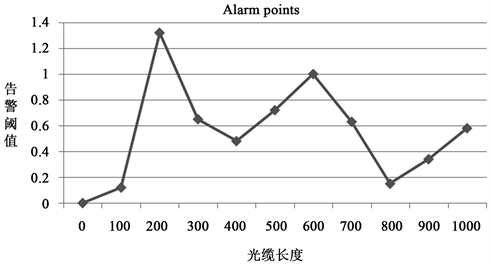Figure 7. The simulation of fiber optic cable fault point

6. 结论

  王小辉, 李圣普, 刘建粉, 等. 光纤传感在电力传输安全监测中的应用研究[J]. 信息技术, 2013(2): 39-41.  Macii, D., Petri, D. and Zorat, A. (2012) Accuracy Analysis and Enhancement of DFT-Based Synchrophasor Estimators in Off-Nominal Conditions. IEEE Transactions on Instrumentation and Measurement, 61, 2653-2664. https://doi.org/10.1109/TIM.2012.2199197  Ren, J.F. and Kezunovic, M. (2011) Real-Time Power System Frequency and Phasors Estimation Using Recursive Wavelet Transform. IEEE Transactions on Power Delivery, 26, 1392-1402. https://doi.org/10.1109/TPWRD.2011.2135385  李川. 光纤传感器技术[M]. 北京: 科学出版社, 2012.  李大虎, 曹一家. 基于模糊滤波和Prony算法的低频振荡模式在线辨识方法[J]. 电力系统自动化, 2007(1): 14-19.  陈文雯, 刘友宽, 孙建平. 群体智能的系统辨识[J]. 云南电力技术. 2014(3): 10-14  丁军策, 蔡泽祥, 李建设, 等. 基于混合量测的电力系统动态状态估计[J]. 南方电网技术, 2009(5): 94-98  刘永莉. 分布式光纤传感技术在边坡工程监测中的应用研究[D]. 杭州: 浙江大学, 2011.  Naruse, H., Uchiyama, Y., Kurashima, T., et al. (2000) River Levee Change Detection Using Distributed Fiber Optic Strain Sensor. IEICE Trans Electron, 83, 462-467.  Kohashi, H., Katou, S., Ando, S., et al. (2004) Application for the Roadway Slope Monitoring Method Using Optical Fiber Sensor. Fujikura Technical Report, 107, 21-27.  李川, 张以谟, 赵永贵. 光纤光栅原理、技术与传感应用[M]. 北京: 科学出版社, 2005.  杨光胤, 葛金. 小波分析故障行波测距法在混合线路中的应用[J]. 云南电力技术, 2008(5): 48-51.  葛飞龙, 肖斌, 高逢顺, 等. 采用光缆线路地物参考点数据表法的故障点定位[J]. 光缆与电缆及其应用技术, 2013(5): 42-44.